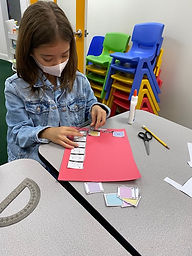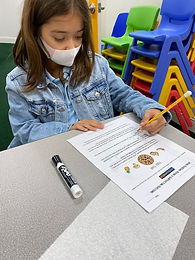## Ms. Dawn

### Target 1​

###### Lesson Type:

Continuation

Geometry

:

Shape

Measure, draw, and classify angles.

###### 1:

Accurately measure angles.

###### 2:

Identify angles as acute, obtuse, or right.

###### 3:

Understand that angles are measured in degrees (from 0 to 360).

###### 4:

Understand that angles have types based on their degree measures (acute, obtuse, and right).

4th

###### Vocabulary:

Acute, Obtuse, Right, Angle, Degrees, Measure, Identify, Protractor

Activities:

1. First, reviewed the definitions of acute, obtuse, and right angle. Also, discussed straight and reflex angle,

2. Students created a storyboard that stated the type of angle each picture represented and used their protractor to measure the angles.

3. Students used grid paper and created their name using their protractor. After creating their name, they measured all the angles in each letter and wrote it in the corresponding area.### Home Exploration

###### Guiding Questions:## Absent Students:

### Target 2

:

###### 1:

Understand the arithmetic process used for adding and subtracting fractions with like denominators.

###### 2:

Simplify the results of an addition or subtraction problem.

###### 3:

Simplify the results of an addition or subtraction problem.

4th

###### Vocabulary:

Fractions, Mixed numbers, CUBES

Activities:

1. Reviewed the acronym CUBES and did a sample word problem together to practice strategy.

2. Students used CUBES to solve other addition and subtraction fraction/mixed number word problems.

3. Students drew pictures on the whiteboard to help show their thinking of how they came up with their answer. After drawing pictures, they wrote equations to match their drawings.### Home Exploration

###### Guiding Questions:### Target 3

:

###### Vocabulary:

Activities:### Home Exploration